# 商品管理系统

## 题干信息

3．创新要求

1．功能描述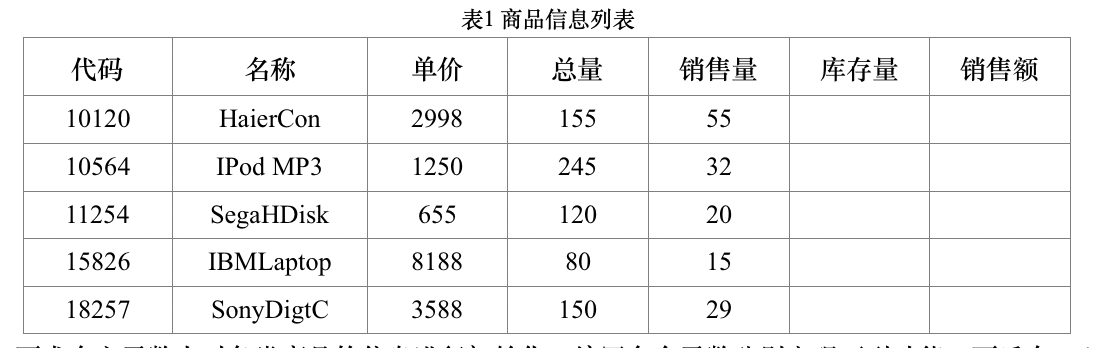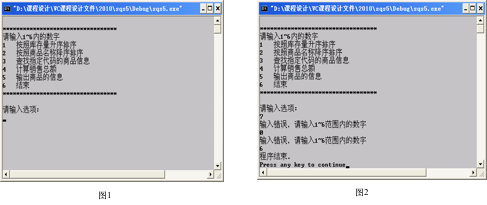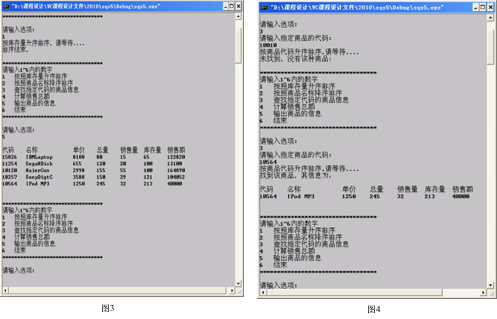## 测试样例

5
10120 HaierCon 2998 155 55
10564 IPodMP3 1250 245 32
11254 SegaHDisk 655 120 20
15826 IBMLapgtop 8188 80 15
18257 SontDigtC 3588 150 29

## 代码

//
//  main.cpp
//  LCS
//
//  Created by 陈冉飞 on 2019/6/21.
//  Copyright © 2019 陈冉飞. All rights reserved.
//

//#include <bits/stdc++.h>
#include<iostream>
#include<iomanip>
using namespace std;
#define name_max_length 100
//宏定义最多的商品数
#define type_total_max 50

struct goods{
int code;
char name[name_max_length];
int num;   //总量
double price;
int sold_num;
int remain_num;
double total;
};

//计算函数cal
void cal(goods g[],int type_total){
for (int i = 0; i<type_total; i++) {
g[i].remain_num = g[i].num - g[i].sold_num;
g[i].total = g[i].sold_num*g[i].price;
}
}

//排序函数1
void sort1(goods g[],int type_total){
int i,j,pos;
goods temgoods;
for(i = 0; i < type_total-1; i++)
{
pos = i;
for(j = i; j < type_total; j++)
{
if(g[pos].remain_num > g[j].remain_num){
pos = j;
}else if(g[pos].remain_num == g[j].remain_num){
if(g[pos].sold_num > g[i].sold_num){
pos = j;
}
}
}
if(pos != i)
{
temgoods = g[i];
g[i] = g[pos];
g[pos] = temgoods;
}
}
}

//排序函数2
void sort2(goods g[],int type_total){
int i,j,pos;
goods temgoods;
for(i = 0; i < type_total-1; i++)
{
pos = i;
for(j = i; j < type_total; j++)
{
if(strcmp(g[pos].name,g[j].name) < 0)
pos = j;
}
if(pos != i)
{
temgoods = g[i];
g[i] = g[pos];
g[pos] = temgoods;
}
}
}

//查询函数
int search(goods g[],int type_total,char temname[]){
for (int i = 0; i<type_total; i++) {
if (strcmp(g[i].name, temname) == 0) {
return i+1;
}
}
return -1;
}

//汇总函数
int total(goods g[],int type_total){
int sum = 0;
for (int i = 0; i<type_total; i++) {
sum+=g[i].total;
}
return sum;
}

//显示函数
void output(goods g[],int type_total){
cout<<"      代码               名称         单价       总量       销售量    库存量    销售额"<<endl;
for (int i = 0; i<type_total; i++) {
cout<<setw(10)<<g[i].code;
printf("%20s",g[i].name);
cout<<setw(10)<<g[i].price<<setw(10)<<g[i].num<<setw(10)<<g[i].sold_num<<setw(10)<<g[i].remain_num<<setw(10)<<g[i].total<<endl;

}
}

//显示菜单函数
void showMenu()
{
cout<<"******************************"<<endl;
cout<<"请输入1—6内的数字"<<endl;
cout<<"1  按照库存量升序排序"<<endl;
cout<<"2  按照商品名称降序排序"<<endl;
cout<<"3  查找指定代码的商品信息"<<endl;
cout<<"4  计算销售总额"<<endl;
cout<<"5  输出商品的信息"<<endl;
cout<<"6  结束"<<endl;
cout<<"******************************"<<endl;
}

int main(int argc, const char * argv[]) {

goods g[type_total_max] = {0};
int type_total;
cout<<"请输入商品种类总数"<<endl;
cin>>type_total;

//输入信息和计算库存量、销售额的部分
for (int i = 0; i<type_total; i++) {
//提示输入
cout<<"请依次输入商品代码、名称、总量、单价、销售量"<<endl;
cin>>g[i].code;
scanf("%s",g[i].name);  //暂时还不能输入有空格的字符串
cin>>g[i].price>>g[i].num>>g[i].sold_num;
}
//调用cal来计算出库存量 销售额
cal(g, type_total);

//成绩管理系统开始
int target_num;
while (true) {
showMenu();
cout<<"请输入选项:"<<endl;
cin>>target_num;
if (target_num<=6&&target_num>=1) {
switch (target_num) {
case 4:
cout<<total(g, type_total)<<endl;
break;
case 5:
output(g, type_total);
break;
case 6:
return 0;
case 1:
sort1(g, type_total);
output(g, type_total);
break;
case 2:
sort2(g, type_total);
output(g, type_total);
break;
case 3:
char target_name[name_max_length];
cout<<"请输入你想查找的名称"<<endl;
scanf("%s",target_name);
int target =search(g,type_total,target_name);
cout<<"      代码               名称         单价       总量       销售量    库存量    销售额"<<endl;
cout<<setw(10)<<g[target].code;
printf("%20s",g[target].name);
cout<<setw(10)<<g[target].price<<setw(10)<<g[target].num<<setw(10);
cout<<g[target].sold_num<<setw(10)<<g[target].remain_num<<setw(10)<<g[target].total<<endl;
break;
}
}
else{
cout<<"输入错误，请输入1-6范围内的数字"<<endl;
}
}
return 0;
}

//
//5
//10120 HaierCon 2998 155 55
//10564 IPodMP3 1250 245 32
//11254 SegaHDisk 655 120 20
//15826 IBMLapgtop 8188 80 15
//18257 SontDigtC 3588 150 29



## 运行结果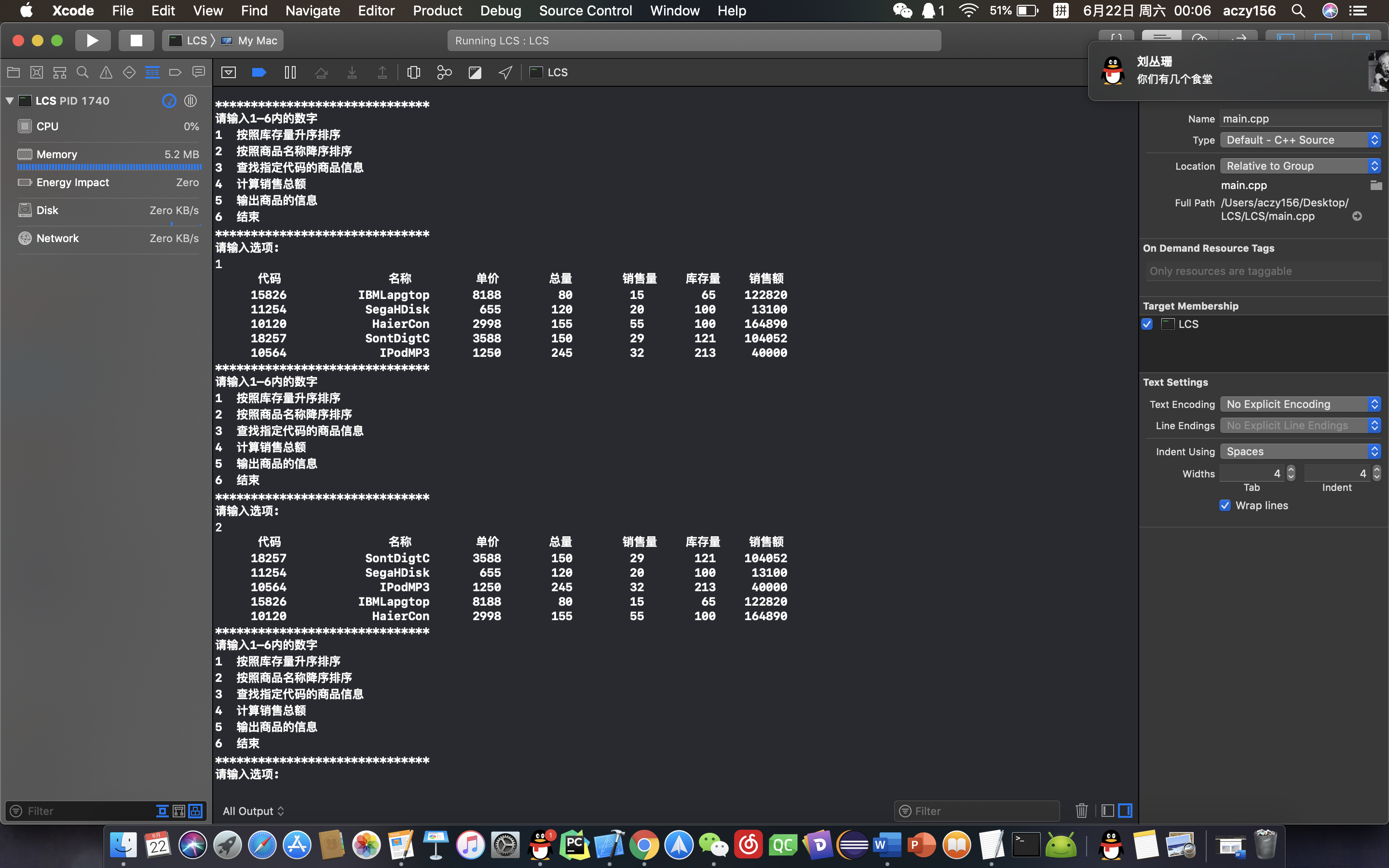# 职工工资信息管理系统

## 题干信息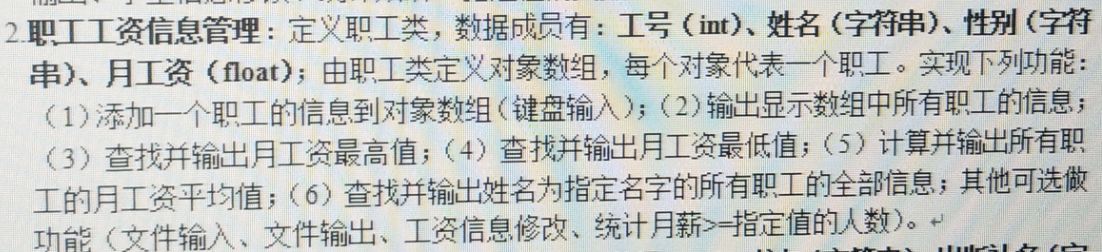## 代码

//
//  main.cpp
//  ZSR
//
//  Created by 陈冉飞 on 2019/6/21.
//  Copyright © 2019 陈冉飞. All rights reserved.
//

//#include <bits/stdc++.h>
#include<iostream>
#include<iomanip>
using namespace std;
#define name_max_length 100
//宏定义最多的商品数
#define type_total_max 50
#define gender_max_length 10

struct goods{
int code;
char name[name_max_length];
char gender[gender_max_length];
float salary;
};

//排序函数1
int sort1(goods g[],int type_total){
int pos = 0,max_salary = -0xffffff;
for (int i = 0; i<type_total; i++) {
if (g[i].salary > max_salary) {
pos = i;
max_salary = g[i].salary;
}
}
return pos+1;
}

//排序函数2
int sort2(goods g[],int type_total){
int pos = 0,min_salary = 0xffffff;
for (int i = 0; i<type_total; i++) {
if (g[i].salary < min_salary) {
pos = i;
min_salary = g[i].salary;
}
}
return pos+1;
}

//查询函数
int search(goods g[],int type_total,char temname[]){
for (int i = 0; i<type_total; i++) {
if (strcmp(g[i].name, temname) == 0) {
return i+1;
}
}
return -1;
}

//汇总函数
int total(goods g[],int type_total){
int sum = 0;
for (int i = 0; i<type_total; i++) {
sum+=g[i].salary;
}
return sum;
}

//显示函数
void output(goods g[],int type_total){
cout<<"      工号               姓名         性别       月工资  "<<endl;
for (int i = 0; i<type_total; i++) {
cout<<setw(10)<<g[i].code;
printf("%20s",g[i].name);
printf("%10s",g[i].gender);
cout<<setw(10)<<g[i].salary<<endl;
}
}

//显示菜单函数
void showMenu()
{
cout<<"******************************"<<endl;
cout<<"请输入1—6内的数字"<<endl;
cout<<"1  显示所有职工的信息"<<endl;
cout<<"2  查找并输出月工资最高值"<<endl;
cout<<"3  查找并输出月工资最低值"<<endl;
cout<<"4  计算并输出所有职工月工资平均值"<<endl;
cout<<"5  查找并输出姓名为制定"<<endl;
cout<<"6  结束"<<endl;
cout<<"******************************"<<endl;
}

int main(int argc, const char * argv[]) {

//文件输入
//    freopen("测试文档名字.txt", "r", stdin);

goods g[type_total_max] = {0};
int type_total;
cout<<"请输入工人总数"<<endl;
cin>>type_total;

//输入信息和计算库存量、销售额的部分
for (int i = 0; i<type_total; i++) {
//提示输入
cout<<"请依次输入工人工号、姓名、性别、月工资"<<endl;
cin>>g[i].code;
scanf("%s",g[i].name);  //暂时还不能输入有空格的字符串
scanf("%s",g[i].gender);
cin>>g[i].salary;
}

//成绩管理系统开始
int target_num;
while (true) {
showMenu();
cout<<"请输入选项:"<<endl;
cin>>target_num;
if (target_num<=6&&target_num>=1) {
switch (target_num) {
case 6:
return 0;
case 1:
output(g, type_total);
break;
case 2:
cout<<"第"<<sort1(g, type_total)<<"个月工资最高"<<endl;
break;
case 3:
cout<<"第"<<sort2(g, type_total)<<"个月工资最低"<<endl;
break;
case 4:
cout<<total(g, type_total)/type_total<<endl;
break;
case 5:
char target_name[name_max_length];
cout<<"请输入你想查找的名称"<<endl;
scanf("%s",target_name);
int target = search(g,type_total,target_name);
cout<<"      工号               姓名         性别       月工资  "<<endl;
cout<<setw(10)<<g[target].code;
printf("%20s",g[target].name);
printf("%10s",g[target].gender);
cout<<setw(10)<<g[target].salary<<endl;
break;
}
}else{
cout<<"输入错误，请输入1-6范围内的数字"<<endl;
}
}
return 0;
}



## 测试样例

6
2635 acb man 7862
2351 asiacjtg women 10923
7812 opaw women 3252
1234 saoiugr women 6880
2365 alsjdgaew men 9023
8971 oierr men 4772

## 运行结果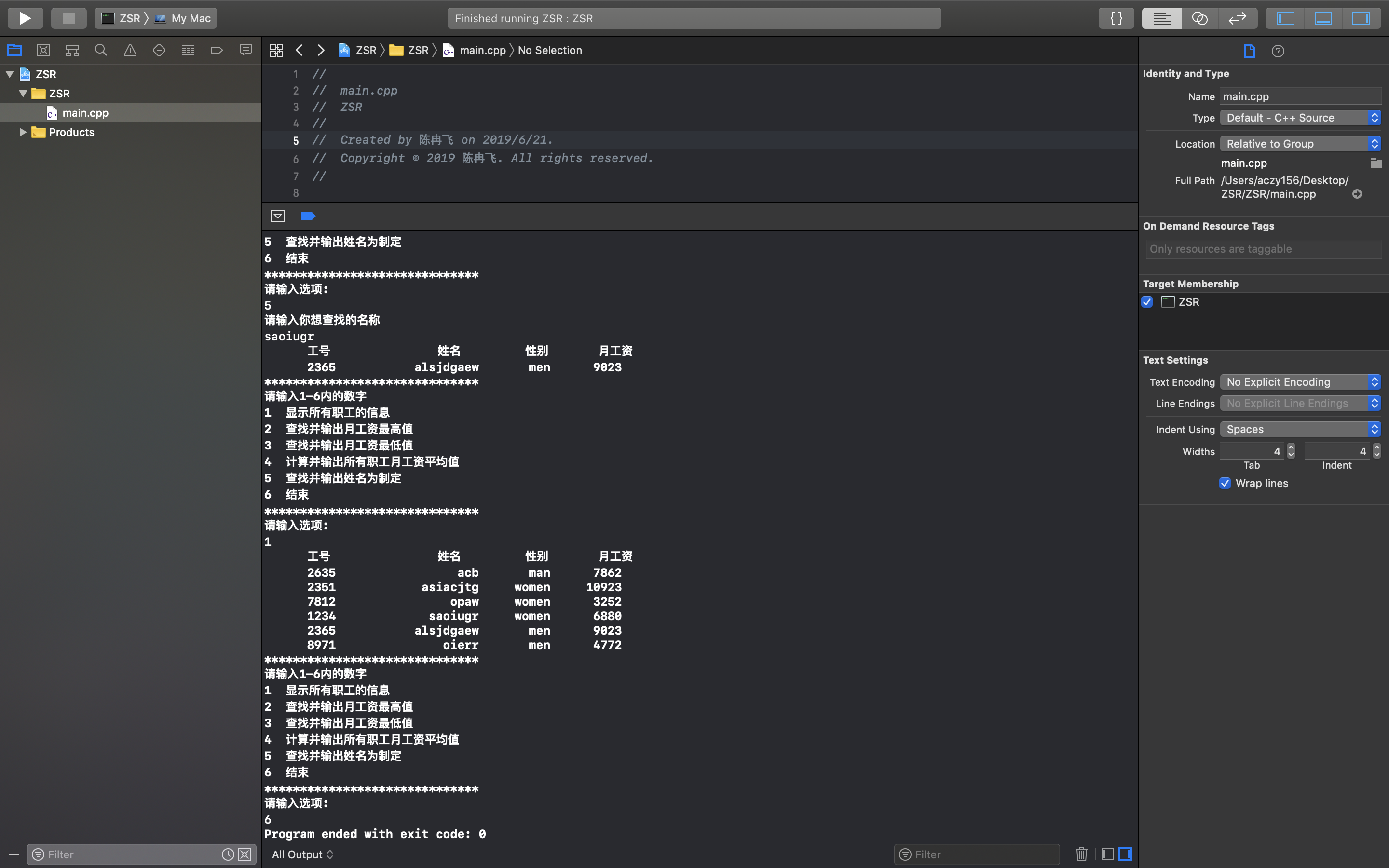# 总结08-26
08-25
07-302240
12-08
10-065430
11-141525
©️2020 CSDN 皮肤主题: 技术工厂 设计师:CSDN官方博客